Identifying Congruent Parts
Congruence Statements
Name that Rule
Mixed Problems
Identify the Rule
100

Given the following statement, which angle is congruent to angle W?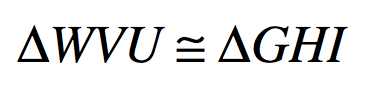Angle G

100

Finish the congruence statement for the two triangles given: △ ABC is Congruent to △ ______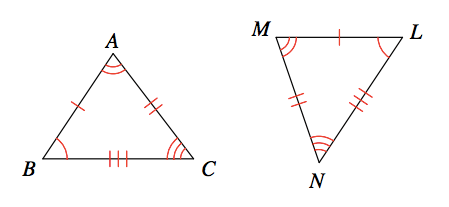MLN

100

Two triangles where we know all three sides are congruent.

Side-Side-Side (SSS)

100

Find the measure of angle F.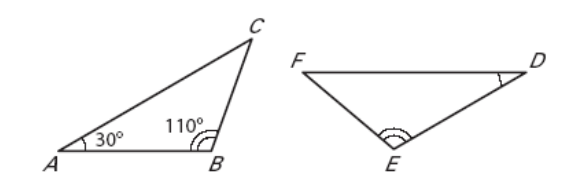40

100

Which rule proves these triangles are congruent?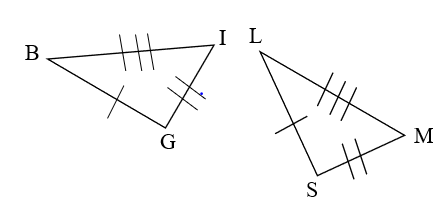SSS test

100

Given the following statement, which side is congruent to VU?HI

100

Finish the congruence statement for the two triangles given: △  EDF is Congruent to △  ______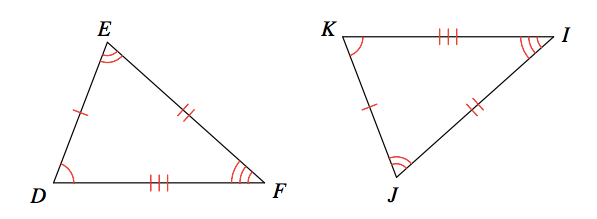JKI

100

Two triangles where we know two angles and the included side are congruent

Angle-Side-Angle (ASA)

100

Given that the triangles are congruent, find the value of x.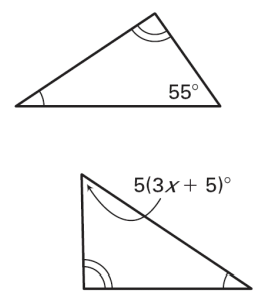x=2

100

Which rule proves these triangles are congruent?SAS test

100

Given the following statement, which side is congruent to IG?UW

100

Finish the congruence statement for the two triangles given: △ BCD is Congruent to △ ______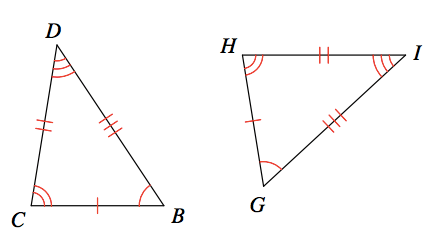GHI

100

Two triangles where we know two sides and the included angle are congruent.

Side-Angle-Side (SAS)

100

Which rule proves these triangles are congruent?AAS

100

Which rule proves these triangles are congruent?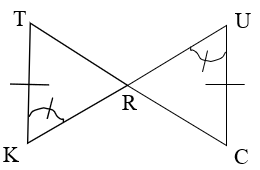AAS

100

Use the diagram to identify which side is congruent to CB.NL

100

Finish the congruence statement for the two triangles given: △ QRP is Congruent to △ ______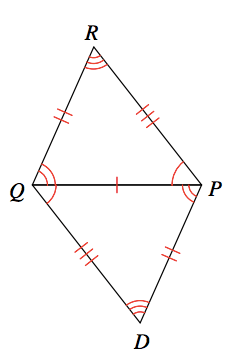PDQ

100

All angles add upto 180 degrees in a triangle

Angle sum property

100

Which are the two possible rules to prove these triangles are congruent where PR and TS are parallel?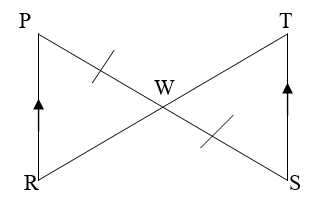AAS or ASA

100

Which rule proves these triangles are congruent?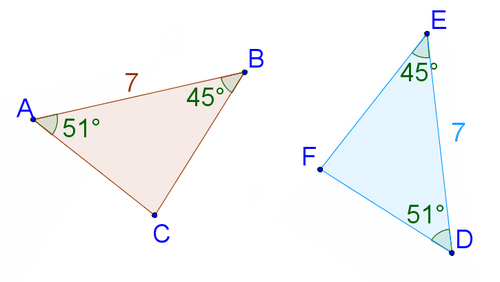ASA test

100

Use the diagram to determine which side is congruent to ML.AB

100

Finish the congruence statement for the two triangles given: △ IRS is Congruent to △  ______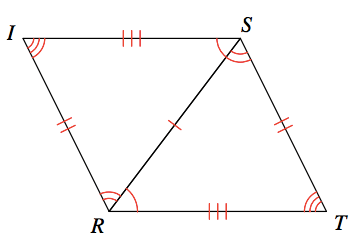TSR

100

Two triangles where we know two angles and the non-included side are congruent.

Angle-Angle-Side (AAS)

100

Which rule proves these triangles are congruent?SSS

100

Which rule proves these triangles are congruent?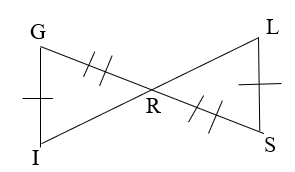No rule to prove congruent

Click to zoom